# 6.5 数据增强-imgaug#

• imgaug的简介和安装

• 使用imgaug对数据进行增强

## 6.5.1 imgaug简介和安装#

### 6.5.1.1 imgaug简介#

imgaug是计算机视觉任务中常用的一个数据增强的包，相比于torchvision.transforms，它提供了更多的数据增强方法，因此在各种竞赛中，人们广泛使用imgaug来对数据进行增强操作。除此之外，imgaug官方还提供了许多例程让我们学习，本章内容仅是简介，希望起到抛砖引玉的功能。

1. Github地址：imgaug

3. 官方提供notebook例程：notebook

### 6.5.1.2 imgaug的安装#

imgaug的安装方法和其他的Python包类似，我们可以通过以下两种方式进行安装

#### conda#

conda config --add channels conda-forge
conda install imgaug


#### pip#

#  install imgaug either via pypi

pip install imgaug

pip install git+https://github.com/aleju/imgaug.git


## 6.5.2 imgaug的使用#

imgaug仅仅提供了图像增强的一些方法，但是并未提供图像的IO操作，因此我们需要使用一些库来对图像进行导入，建议使用imageio进行读入，如果使用的是opencv进行文件读取的时候，需要进行手动改变通道，将读取的BGR图像转换为RGB图像。除此以外，当我们用PIL.Image进行读取时，因为读取的图片没有shape的属性，所以我们需要将读取到的img转换为np.array()的形式再进行处理。因此官方的例程中也是使用imageio进行图片读取。

### 单张图片处理#

import imageio
import imgaug as ia
%matplotlib inline

# 图片的读取

# 使用Image进行读取
# img = Image.open("./Lenna.jpg")
# image = np.array(img)
# ia.imshow(image)

# 可视化图片
ia.imshow(img)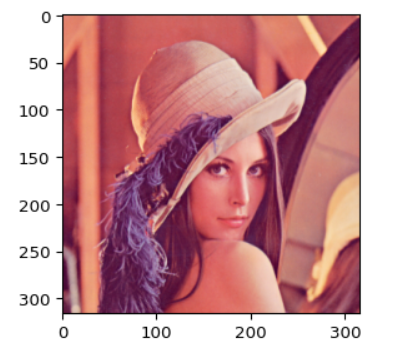from imgaug import augmenters as iaa

# 设置随机数种子
ia.seed(4)

# 实例化方法
rotate = iaa.Affine(rotate=(-4,45))
img_aug = rotate(image=img)
ia.imshow(img_aug)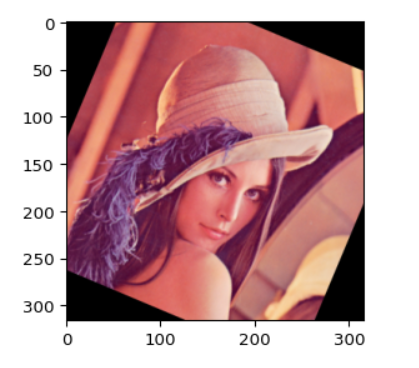iaa.Sequential(children=None, # Augmenter集合
random_order=False, # 是否对每个batch使用不同顺序的Augmenter list
name=None,
deterministic=False,
random_state=None)

# 构建处理序列
aug_seq = iaa.Sequential([
iaa.Affine(rotate=(-25,25)),
iaa.Crop(percent=(0,0.2))
])
# 对图片进行处理，image不可以省略，也不能写成images
image_aug = aug_seq(image=img)
ia.imshow(image_aug)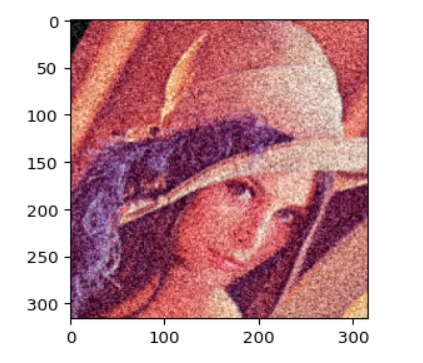### 对批次图片进行处理#

#### 对批次的图片以同一种方式处理#

images = [img,img,img,img,]
images_aug = rotate(images=images)
ia.imshow(np.hstack(images_aug))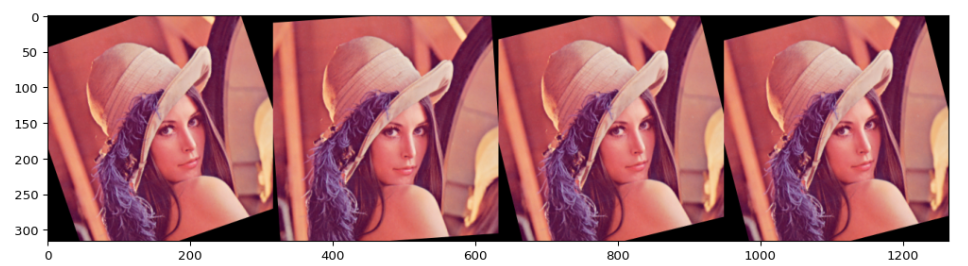在上述的例子中，我们仅仅对图片进行了仿射变换，同样的，我们也可以对批次的图片使用多种增强方法，与单张图片的方法类似，我们同样需要借助Sequential来构造数据增强的pipline。

aug_seq = iaa.Sequential([
iaa.Affine(rotate=(-25, 25)),
iaa.Crop(percent=(0, 0.2))
])

# 传入时需要指明是images参数
images_aug = aug_seq.augment_images(images = images)
#images_aug = aug_seq(images = images)
ia.imshow(np.hstack(images_aug))


#### 对批次的图片分部分处理#

imgaug相较于其他的数据增强的库，有一个很有意思的特性，即就是我们可以通过imgaug.augmenters.Sometimes()对batch中的一部分图片应用一部分Augmenters,剩下的图片应用另外的Augmenters。

iaa.Sometimes(p=0.5,  # 代表划分比例
then_list=None,  # Augmenter集合。p概率的图片进行变换的Augmenters。
else_list=None,  #1-p概率的图片会被进行变换的Augmenters。注意变换的图片应用的Augmenter只能是then_list或者else_list中的一个。
name=None,
deterministic=False,
random_state=None)


### 对不同大小的图片进行处理#

# 构建pipline
seq = iaa.Sequential([
iaa.AddToHueAndSaturation((-60, 60)),  # change their color
iaa.ElasticTransformation(alpha=90, sigma=9),  # water-like effect
iaa.Cutout()  # replace one squared area within the image by a constant intensity value
], random_order=True)

# 加载不同大小的图片
images_different_sizes = [
]

# 对图片进行增强
images_aug = seq(images=images_different_sizes)

# 可视化结果
print("Image 0 (input shape: %s, output shape: %s)" % (images_different_sizes.shape, images_aug.shape))
ia.imshow(np.hstack([images_different_sizes, images_aug]))

print("Image 1 (input shape: %s, output shape: %s)" % (images_different_sizes.shape, images_aug.shape))
ia.imshow(np.hstack([images_different_sizes, images_aug]))

print("Image 2 (input shape: %s, output shape: %s)" % (images_different_sizes.shape, images_aug.shape))
ia.imshow(np.hstack([images_different_sizes, images_aug]))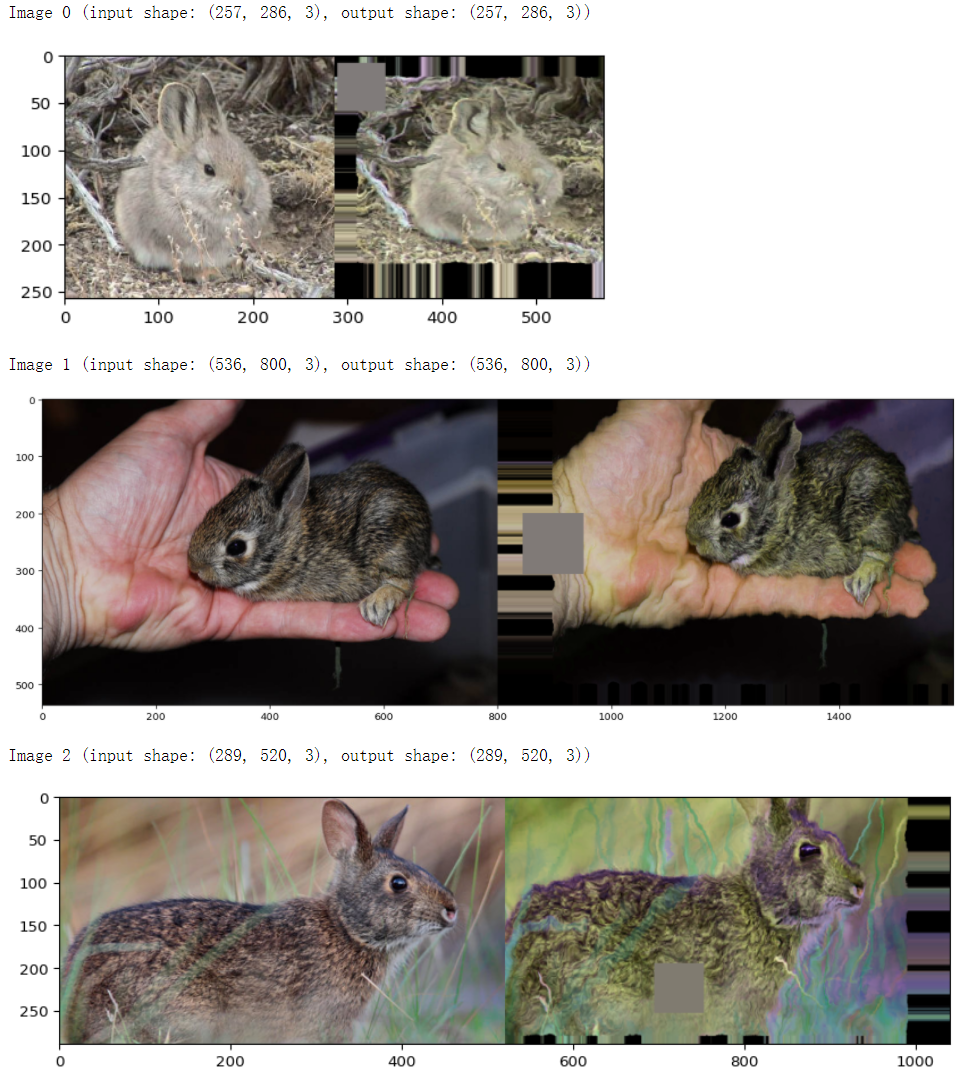## 6.5.3 imgaug在PyTorch的应用#

import numpy as np
from imgaug import augmenters as iaa
from torchvision import transforms

# 构建pipline
tfs = transforms.Compose([
iaa.Sequential([
iaa.flip.Fliplr(p=0.5),
iaa.flip.Flipud(p=0.5),
iaa.GaussianBlur(sigma=(0.0, 0.1)),
iaa.MultiplyBrightness(mul=(0.65, 1.35)),
]).augment_image,
# 不要忘记了使用ToTensor()
transforms.ToTensor()
])

# 自定义数据集
class CustomDataset(Dataset):
def __init__(self, n_images, n_classes, transform=None):
# 图片的读取，建议使用imageio
self.images = np.random.randint(0, 255,
(n_images, 224, 224, 3),
dtype=np.uint8)
self.targets = np.random.randn(n_images, n_classes)
self.transform = transform

def __getitem__(self, item):
image = self.images[item]
target = self.targets[item]

if self.transform:
image = self.transform(image)

return image, target

def __len__(self):
return len(self.images)

def worker_init_fn(worker_id):
imgaug.seed(np.random.get_state() + worker_id)

custom_ds = CustomDataset(n_images=50, n_classes=10, transform=tfs)